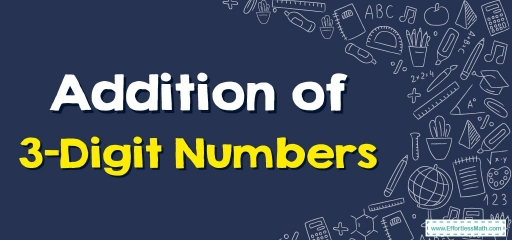Adding three-digit numbers is very simple and we will teach you it in a few steps in this article.After the number $$99$$, the counting numeral is $$100$$. That’s a $$3$$-digit number. Whenever one gets added with $$99$$, the answer is $$100$$.

Three-digit numbers begin at $$100$$ and then proceed to $$999$$. Then, you get into the thousands (or four-digit numbers). The addition of two $$3$$-digit numbers takes several steps.

## How are three-digit numbers added?

Step one: Put one number over the other number so the hundreds, tens, and ones spot all line up. Place a plus sign $$(+)$$ to the left of these numbers. As an alternative to the equal sign $$(=)$$, place a line under the lower figure.

Note: Whenever addition expressions are stacked, be certain these numbers end up lined up properly. These numbers ought to always get lined up on the right. It doesn’t matter the quantity of these numbers you are adding have, the ones on the right always get lined up.

Step two: Add digits in the one’s spot. If that numeral is higher than $$10$$  put a one above the tens spot column and put the ones’ under the line in the one’s spot column.

Step three: Add the digits in the tens spot and put this answer under the line and in the tens spot column.

Step four: Add the numerals in the hundreds spot column and put this answer under the line and before the other numeral under that line.

As you see, problems involving stacked always get solved from right to left. That means you must always begin by adding the digits that are on the right.

### Addition of 3-Digit Numbers – Example 1:

Find the sum.

$$\begin{array}{r} &352\\ +\!\!\!\!\!&135\\ \hline \end{array}$$

Solution:

First line up the numbers: $$\begin{array}{r} &352\\ +\!\!\!\!\!&135\\ \hline \end{array}→$$ Add the numerals in the ones’ spot column $$(2 + 5 = 7)$$ and put the resulting answer under the line. $$\begin{array}{r} &352\\ +\!\!\!\!\!\!&135 \\ \hline \end{array} \\ \ \ \ \ \ \ \ \ \ \ \ 7$$ , Continue with tens’ place. $$5 \ + \ 3=8$$, $$\begin{array}{r} &352\\ +\!\!\!\!\!\!&135 \\ \hline \end{array} \\ \ \ \ \ \ \ \ \ 8$$. Add the numerals in the hundreds’ spot column then put this answer under the line. $$3 \ + \ 1=4$$, $$\begin{array}{r} &352\\ +\!\!\!\!\!\!&135 \\ \hline \end{array} \\ \ \ \ \ \ \ 487$$

### Addition of 3-Digit Numbers – Example 2:

Find the sum.

$$\begin{array}{r} &273\\ +\!\!\!\!\!&432\\ \hline \end{array}$$

Solution:

First line up the numbers: $$\begin{array}{r} &273\\ +\!\!\!\!\!&432\\ \hline \end{array}→$$ Add the numerals in the ones’ spot column $$(3 + 2 = 5)$$ and put the resulting answer under the line. $$\begin{array}{r} &273\\ +\!\!\!\!\!\!&432 \\ \hline \end{array} \\ \ \ \ \ \ \ \ \ \ \ \ 5$$ , Continue with tens’ place. $$7 \ + \ 3=10$$. The numeral is $$10$$  put a one above the tens spot column and put the $$0$$ under the line in the one’s spot column. $$\begin{array}{r} &273\\ +\!\!\!\!\!\!&432 \\ \hline \end{array} \\ \ \ \ \ \ \ \ \ 0$$. Add the numerals in the hundreds’ spot column then put this answer under the line. Whilst adding the numbers in the hundreds column, you also add the tens number which you carried forward from your one’s $$2 + 4+1=7$$, $$\begin{array}{r} &273\\ +\!\!\!\!\!\!&432 \\ \hline \end{array} \\ \ \ \ \ \ \ 705$$

## Exercises for Addition of 3-Digit Numbers

1. $$\color{blue}{750+224}$$
2. $$\color{blue}{820+170}$$
3. $$\color{blue}{445+352}$$
4. $$\color{blue}{634+315}$$
1. $$\color{blue}{974}$$
2. $$\color{blue}{990}$$
3. $$\color{blue}{797}$$
4. $$\color{blue}{949}$$

### What people say about "Addition of 3-Digit Numbers - Effortless Math: We Help Students Learn to LOVE Mathematics"?

No one replied yet.

X
30% OFF

Limited time only!

Save Over 30%

SAVE $5 It was$16.99 now it is \$11.99